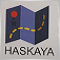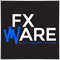# EA is closing trades of other EA1264

Hi all,

I am recently testing an EA that I developed myself and I found that for some reason, when it closes a position on a pair, it closes all other positions in all other pairs, regardless if the EA is running on that pair.

For example, if the EA is running on EURJPY and one condition is met for closing the trades on the EURJPY pair, the EA closes not only those trades, but all other trades that are opened in any pair, even if it is not loaded in that chart.

I checked if the EA could be sharing the magic number between other EA but its not the case.

Here is the code responsible of closing the trades and removing pending orders.

Any help would be appreciated!

Thank you!

```void checkTrades()
{
for(int i=OrdersTotal()-1;i>=0;i--)
{
{
{
if(SellCheck(1) && closeOn0)
{
removePending();
double totalLots=OrderLots();
if(OrderClose(OrderTicket(),totalLots,Bid,Slippage,0))
{
Print("****Condition 1*****");
}
return;
}

if(SellCheck(1) && closeOn1)
{
removePending();
double totalLots=OrderLots();
if(OrderClose(OrderTicket(),totalLots,Bid,Slippage,0))
{
Print("****Condition 1*****");
}
return;
}

if(stop==1 && iClose(_Symbol,PERIOD_D1,1)<Limit)
{
removePending();
double totalLots=OrderLots();
if(OrderClose(OrderTicket(),totalLots,Bid,Slippage,0))
{
Print("*****Condition 2*****");
}
return;
}
}
if(OrderSymbol()==Symbol() && OrderMagicNumber()==Magic && OrderType()==OP_SELL)
{

{
removePending();
double totalLots=OrderLots();
{
Print("****Condition 0*****");
}
return;
}

{
removePending();
double totalLots=OrderLots();
{
Print("****Condition 1*****");
}
return;
}

if(stop==1 && iClose(_Symbol,PERIOD_D1,1)>Limit)
{
removePending();
double totalLots=OrderLots();
{
Print("*****Condition 2*****");
}
return;
}
}
}
}
}

void removePending()
{
for(int i=OrdersTotal()-1;i>=0;i--)
{
{
if(OrderSymbol()==Symbol() && OrderMagicNumber()==Magic && (OrderType()==OP_BUYLIMIT || OrderType()==OP_SELLLIMIT))
{
OrderDelete(OrderTicket(),0);
}
}
}
}```2044

ppsev:

Hi all,

I am recently testing an EA that I developed myself and I found that for some reason, when it closes a position on a pair, it closes all other positions in all other pairs, regardless if the EA is running on that pair.

For example, if the EA is running on EURJPY and one condition is met for closing the trades on the EURJPY pair, the EA closes not only those trades, but all other trades that are opened in any pair, even if it is not loaded in that chart.

I checked if the EA could be sharing the magic number between other EA but its not the case.

Here is the code responsible of closing the trades and removing pending orders.

Any help would be appreciated!

Thank you!

```//+------------------------------------------------------------------+
{
for(int i=OrdersTotal()-1;i>=0;i--)
{
{
{
if(SellCheck(1) && closeOn0) // OrderSymbol()==Symbol() && OrderMagicNumber()==Magic
{
removePending();
double totalLots=OrderLots();
if(OrderClose(OrderTicket(),totalLots,Bid,Slippage,0))
{
Print("****Condition 1*****");
}
return;
}

if(SellCheck(1) && closeOn1)// OrderSymbol()==Symbol() && OrderMagicNumber()==Magic
{
removePending();
double totalLots=OrderLots();
if(OrderClose(OrderTicket(),totalLots,Bid,Slippage,0))
{
Print("****Condition 1*****");
}
return;
}

if(stop==1 && iClose(_Symbol,PERIOD_D1,1)<Limit) OrderSymbol()==Symbol() && OrderMagicNumber()==Magic
{
removePending();
double totalLots=OrderLots();
if(OrderClose(OrderTicket(),totalLots,Bid,Slippage,0))
{
Print("*****Condition 2*****");
}
return;
}
}
if(OrderSymbol()==Symbol() && OrderMagicNumber()==Magic && OrderType()==OP_SELL)
{

if(BuyCheck(1) && closeOn0) OrderSymbol()==Symbol() && OrderMagicNumber()==Magic
{
removePending();
double totalLots=OrderLots();
{
Print("****Condition 0*****");
}
return;
}

if(BuyCheck(1) && closeOn1) OrderSymbol()==Symbol() && OrderMagicNumber()==Magic
{
removePending();
double totalLots=OrderLots();
{
Print("****Condition 1*****");
}
return;
}

if(stop==1 && iClose(_Symbol,PERIOD_D1,1)>Limit) OrderSymbol()==Symbol() && OrderMagicNumber()==Magic
{
removePending();
double totalLots=OrderLots();
{
Print("*****Condition 2*****");
}
return;
}
}
}
}
}

void removePending()
{
for(int ix=OrdersTotal()-1;ix>=0;ix--)
{
{
if(OrderSymbol()==Symbol() && OrderMagicNumber()==Magic && (OrderType()==OP_BUYLIMIT || OrderType()==OP_SELLLIMIT))
{
OrderDelete(OrderTicket(),0);
}
}
}
}```1264

Mehmet Bastem:

I don't get it. You suggest to change the structure of the function and put the magic number and symbol with the other conditions?

If this is the case, why it makes a difference with the actual function? I mean, if one of the conditions in the "if" statement aren't meeted, then the trades in other pairs shouldn't be touched.

Why this happens?1264

Hi,

I did this changes, but the results are the same. I don't know what I'm doing wrong.

```void checkTrades()
{
for(int i=OrdersTotal()-1;i>=0;i--)
{
{
{
if(SellCheck(1) && closeOn0 && OrderSymbol()==Symbol() && OrderMagicNumber()==Magic)
{
removePending();
double totalLots=OrderLots();
if(OrderClose(OrderTicket(),totalLots,Bid,Slippage,0))
{
Print("****Condition 1*****");
}
return;
}

if(SellCheck(1) && closeOn1)// OrderSymbol()==Symbol() && OrderMagicNumber()==Magic
{
removePending();
double totalLots=OrderLots();
if(OrderClose(OrderTicket(),totalLots,Bid,Slippage,0))
{
Print("****Condition 1*****");
}
return;
}

if(stop==1 && iClose(_Symbol,PERIOD_D1,1)<Limit && OrderSymbol()==Symbol() && OrderMagicNumber()==Magic)
{
removePending();
double totalLots=OrderLots();
if(OrderClose(OrderTicket(),totalLots,Bid,Slippage,0))
{
Print("*****Condition 2*****");
}
return;
}
}
if(/*OrderSymbol()==Symbol() && OrderMagicNumber()==Magic && */OrderType()==OP_SELL)
{

if(BuyCheck(1) && closeOn0 && OrderSymbol()==Symbol() && OrderMagicNumber()==Magic)
{
removePending();
double totalLots=OrderLots();
{
Print("****Condition 0*****");
}
return;
}

if(BuyCheck(1) && closeOn1 && OrderSymbol()==Symbol() && OrderMagicNumber()==Magic)
{
removePending();
double totalLots=OrderLots();
{
Print("****Condition 1*****");
}
return;
}

if(stop==1 && iClose(_Symbol,PERIOD_D1,1)>Limit && OrderSymbol()==Symbol() && OrderMagicNumber()==Magic)
{
removePending();
double totalLots=OrderLots();
{
Print("*****Condition 2*****");
}
return;
}
}
}
}
}
//+------------------------------------------------------------------+
//|                                                                  |
//+------------------------------------------------------------------+
void removePending()
{
for(int i=OrdersTotal()-1;i>=0;i--)
{
{
if(OrderSymbol()==Symbol() && OrderMagicNumber()==Magic && (OrderType()==OP_BUYLIMIT || OrderType()==OP_SELLLIMIT))
{
OrderDelete(OrderTicket(),0);
}
}
}
}```9204

ppsev:

Hi,

I did this changes, but the results are the same. I don't know what I'm doing wrong.

Hi, try check this logic..

Print(OrderSymbol()==Symbol() && OrderMagicNumber()==Magic);

Your routine go thru this logic is not possible. Or you may confusing with a file to run and file to code is save in different location.   Chaiya471

ppsev:

Hi,

I did this changes, but the results are the same. I don't know what I'm doing wrong.

You are calling OrderSelect() in nested loops. You have first loop in checkTrades() and then you call second loop in removePending() and that is overwriting order selected by the first loop because only one order can be selected at a time.1264

You are calling OrderSelect() in nested loops. You have first loop in checkTrades() and then you call second loop in removePending() and that is overwriting order selected by the first loop because only one order can be selected at a time.

Ohh, so if I don't use a function to remove the pending orders and just remove them in the checkTrades loop, that would fix the problem?

Or I should execute removePending() if one of the 3 conditiones are met for closing a trade?471

ppsev:

Ohh, so if I don't use a function to remove the pending orders and just remove them in the checkTrades loop, that would fix the problem?

The problem is not that you are using a function but that this function contains a loop with OrderSelect(). Don't use nested loops and you'll be fine.471

ppsev:

Ohh, so if I don't use a function to remove the pending orders and just remove them in the checkTrades loop, that would fix the problem?

Or I should execute removePending() if one of the 3 conditiones are met for closing a trade?

Untested quick fix of your code to avoid this and preserve logic

```if(checkTrades()) removePending();
//---

{
for(int i=OrdersTotal()-1;i>=0;i--)
{
{
{
if(SellCheck(1) && closeOn0 && OrderSymbol()==Symbol() && OrderMagicNumber()==Magic)
{
double totalLots=OrderLots();
if(OrderClose(OrderTicket(),totalLots,Bid,Slippage,0))
{
Print("****Condition 1*****");
}
return (true);
}

if(SellCheck(1) && closeOn1)// OrderSymbol()==Symbol() && OrderMagicNumber()==Magic
{
double totalLots=OrderLots();
if(OrderClose(OrderTicket(),totalLots,Bid,Slippage,0))
{
Print("****Condition 1*****");
}
return (true);
}

if(stop==1 && iClose(_Symbol,PERIOD_D1,1)<Limit && OrderSymbol()==Symbol() && OrderMagicNumber()==Magic)
{
double totalLots=OrderLots();
if(OrderClose(OrderTicket(),totalLots,Bid,Slippage,0))
{
Print("*****Condition 2*****");
}
return (true);
}
}
if(/*OrderSymbol()==Symbol() && OrderMagicNumber()==Magic && */OrderType()==OP_SELL)
{

if(BuyCheck(1) && closeOn0 && OrderSymbol()==Symbol() && OrderMagicNumber()==Magic)
{
double totalLots=OrderLots();
{
Print("****Condition 0*****");
}
return (true);
}

if(BuyCheck(1) && closeOn1 && OrderSymbol()==Symbol() && OrderMagicNumber()==Magic)
{
double totalLots=OrderLots();
{
Print("****Condition 1*****");
}
return (true);
}

if(stop==1 && iClose(_Symbol,PERIOD_D1,1)>Limit && OrderSymbol()==Symbol() && OrderMagicNumber()==Magic)
{
double totalLots=OrderLots();
{
Print("*****Condition 2*****");
}
return (true);
}
}
}
}
return (false);
}
//---

void removePending()
{
for(int i=OrdersTotal()-1;i>=0;i--)
{
{
if(OrderSymbol()==Symbol() && OrderMagicNumber()==Magic && (OrderType()==OP_BUYLIMIT || OrderType()==OP_SELLLIMIT))
{
OrderDelete(OrderTicket(),0);
}
}
}
}
//+------------------------------------------------------------------+
```1264